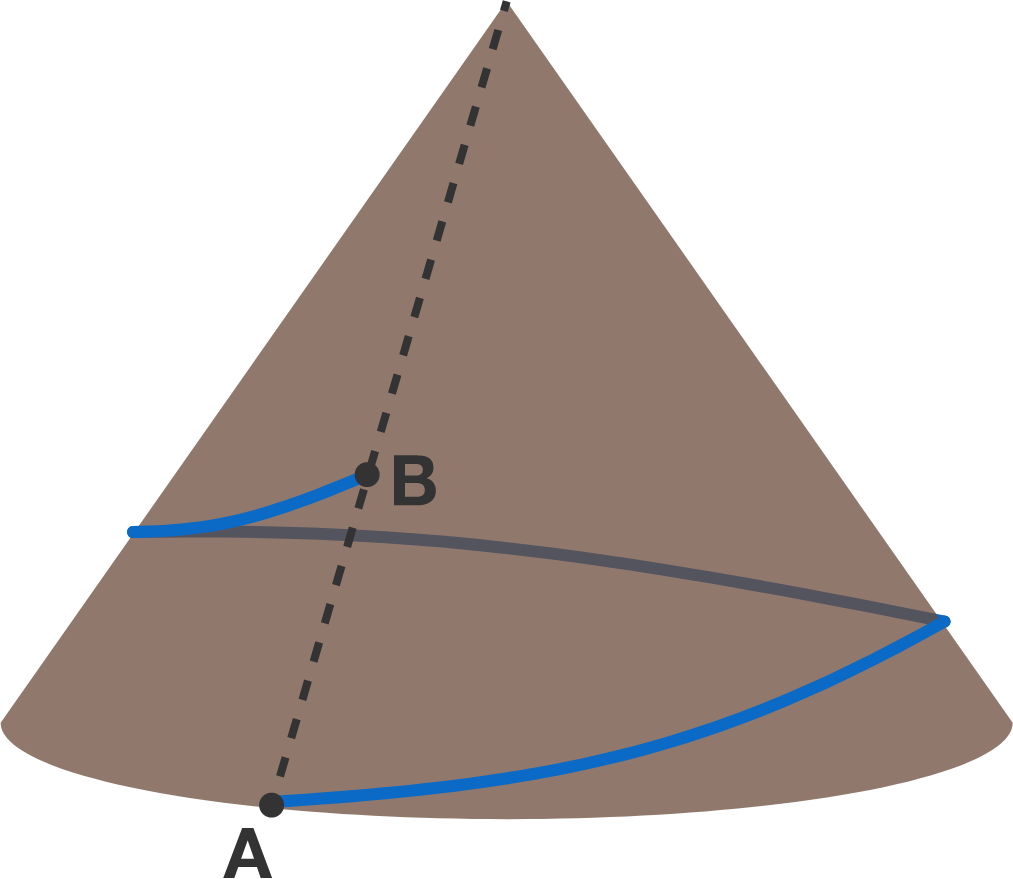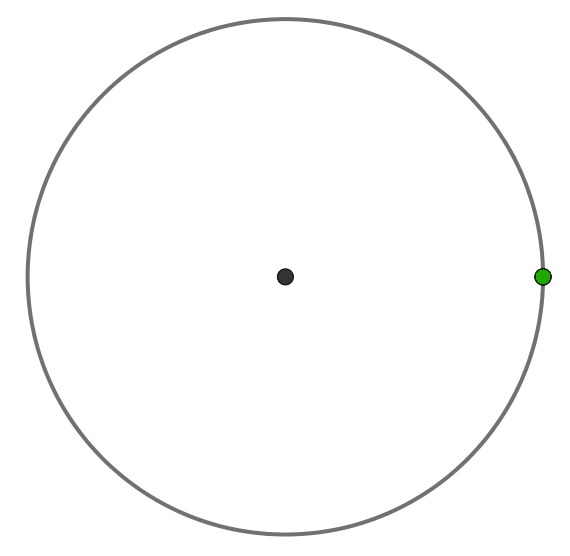# Problems of the Week

Contribute a problem

# 2018-04-09 AdvancedThe parks service would like to construct a path that starts at point A and revolves around the mountain once before ending at point B.

Which describes the uphill/downhill nature of the shortest possible path?

Note: Point A is at the base of a mountain in the shape of a right circular cone. Point B is located on the segment between point A and the summit.

Let $$f,g : \mathbb{R} \longrightarrow \mathbb{R}$$ such that $$f(2x)=2f(x)$$ and $$g(x) = x+ f\big(g(x)\big).$$

If $$2g(x)$$ is in the range of $$g$$ for all real $$x,$$ then is it always true that $$g(2x)=2g(x)?$$

Note: $$f$$ and $$g$$ are not necessarily continuous over $$\mathbb{R}.$$

The rate of a simple chemical reaction like $$\ce{A + 2B->C}$$ depends on the input concentrations in a simple way. Here, for example, the output species $$\text{C}$$ is made with a rate proportional to $$\left[\text{A}\right]\cdot\left[\text{B}\right]^2$$ and, in general, the rates of one step reactions depend on the input concentrations raised to the power of their stoichiometric coefficient. But this isn't always the case.

Chain reactions occur when the output from one reaction $$\mathcal{R}_i$$ is the input to another $$\mathcal{R}_j$$ as in the following system of reactions: $\begin{array}{rrr} \text{A} &\longleftrightarrow& \text{2B } \\ \text{B} &\longleftrightarrow& \text{3C } \\\ \text{C} &\longleftrightarrow& \text{4D } \\ \text{D} &\longrightarrow& \text{E}. \end{array}$ It's a lot less simple to find reaction order in a case like this, but as the concentration of $$\text{A}$$ rises, a simple behavior emerges: in the asymptotic limit where $$\left[\text{A}\right]$$ gets large, the rate of production of the output $$\left[\text{E}\right]$$ is approximately equal to $$\text{(a constant)}\times\left[\text{A}\right]^\alpha.$$

What is $$\alpha?$$

Assume that fresh $$\text{A}$$ is supplied continuously to the system so that its concentration is always $$\left[\text{A}_0\right],$$ and that the conversion of $$\text{D}$$ to $$\text{E}$$ is irreversible. For simplicity, assume all the magnitudes of all rate constants are equal to $$1.$$

$\begin{array}{lrr} &&A & B \\ -&&C& D \\ \hline \\ &&E & F \\ + &&G& H\\ \hline \\ &P&P& P \end{array}$

Each of the letters $$A, B, C, D, E, F, G, H, P$$ represents a distinct digit from 1 to 9.

What is $$A+B+C+D?$$

Three points $${\color{blue}A}, {\color{red}B},$$ and $${\color{green}C}$$ are moving counterclockwise around a unit circle with periods $$\SI{1}{\second}, \SI{2}{\second},$$ and $$\SI{3}{\second},$$ respectively.

They started from the same position on the circle.

What is the maximal area of triangle $${\color{blue}A}{\color{red}B}{\color{green}C}?$$×

Problem Loading...

Note Loading...

Set Loading...